# Cap

Jesters hat is shaped a rotating cone. Calculate how much paper is needed to the cap 60 cm high when head circumference is 52 cm.

Result

S =  1574.8 cm2

#### Solution:Leave us a comment of example and its solution (i.e. if it is still somewhat unclear...):Be the first to comment!#### To solve this example are needed these knowledge from mathematics:

Pythagorean theorem is the base for the right triangle calculator. Do you want to convert length units?

## Next similar examples:

1. Area of the cone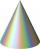Calculate the surface area of the cone, you know the base diameter 25 cm and a height 40 cm.
2. Observatory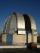Observatory dome has the shape of a hemisphere with a diameter d = 10 m. Calculate the surface.
3. The diagram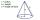The diagram is a cone of radius 8cm and height 10cm. The diameter of the base is. ..
4. CablewayCableway has a length of 1800 m. The horizontal distance between the upper and lower cable car station is 1600 m. Calculate how much meters altitude is higher upper station than the base station.
5. Athlete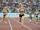How long length run athlete when the track is circular shape of radius 120 meters and an athlete runs five times in the circuit?
6. Four ropesTV transmitter is anchored at a height of 44 meters by four ropes. Each rope is attached at a distance of 55 meters from the heel of the TV transmitter. Calculate how many meters of rope were used in the construction of the transmitter. At each attachment.
7. Chord 4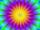I need to calculate the circumference of a circle, I know the chord length c=22 cm and the distance from the center d=29 cm chord to the circle.
8. Circle r,DCalculate the diameter and radius of the circle if it has length 52.45 cm.
9. Bicycle wheelAfter driving 157 m bicycle wheel rotates 100 times. What is the radius of the wheel in cm?
10. MineWheel in traction tower has a diameter 5 m. How many meters will perform an elevator cabin if wheel rotates in the same direction 49 times?
11. CircleWhat is the radius of the circle whose perimeter is 6 cm?
12. Clock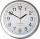How long is trajectory of second hand of hours for day, if is 15 mm long?
13. FlowerbedIn the park there is a large circular flowerbed with a diameter of 12 m. Jakub circulated him ten times and the smaller Vojtoseven times. How many meters each went by and how many meters did Jakub run more than Vojta?
14. Drawer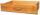The rectangular face of a drawer has a perimeter of 108 cm and a width of 18cm. Find the length and the area of the rectangular face of the drawer.
15. Circle - simpleThe circumference of a circle is 930 mm. How long in mm is its diameter?
16. Clock handsThe second hand has a length of 1.5 cm. How long does the endpoint of this hand travel in one day?
17. Wheel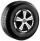What is the wheel diameter if on the 0.38 km track turns 128 times?